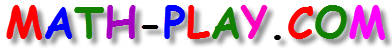#Are you looking for free 6th grade math games? These fun games offer an opportunity for kids to enjoy themselves while reviewing important math concepts.

Here are some important 6th grade math topics:

Factors and Multiples

This is an entertaining math jeopardy game about factors, multiples, prime factorization, GCF (greatest common factor), and LCM (least common multiple).

GCF Jeopardy Game

Find the Greatest Common Factor for a set of numbers in this jeopardy style math game.

One-Step Equations Math Racing Game

Solve one-step equations in this fast-paced, realistic One-Step Equations Math Racing Game.

Properties of Operations Math Racing Game

Identify the properties of equivalent expressions in this Properties of Operations Math Racing Game. Fast-paced, realistic action makes this cool car racing game a winner.

Multiply Decimals Halloween Math Game (New)

In this neat little Multiply Decimals Halloween Math Game, test your math skills about multiplying decimals and have fun zapping spooky monsters. For each correct answer to a math problem, you will enter a 30-second bonus round.

Factors

This millionaire game is a fun way of assessing students' knowledge about factors and divisibility. It can be used as a review activity in the classroom or at home.

Math Magician Integers Game

Is the solution positive, negative, or zero? Try this fun Math Magician Integers Game.

6th Grade Halloween Math Game (New)

Students can have a great time playing this online halloween math game. For each correct answer, you will enter a bonus round where you can earn points by smashing monsters. The math problems are about finding the greatest common factors and the least common multiples.

Math Magician Compare Fractions Game

Have a great time tackling these comparing fractions problems with this exciting Math Magician Compare Fractions Game.

Prime Factorization Spinner Game

In this interactive multi-player game, 6th grade students will practice finding the prime factorization of different composite numbers.

Place Value

This is an interactive millionaire game about place value in whole numbers and decimals. You can play it alone, with a partner, or in two teams.

Making 24 - The Game of Numbers (New)

In this activity, 6th grade students will have to use four whole numbers and different operations to get number 24 as the final answer.

Simplifying Fractions

In this soccer math game kids will have fun reducing fractions to the simplest form.

Reducing Fractions

Do you love baseball? Then play this fun game and practice hitting homeruns and simplifying fractions to earn tons of points.

This is an interactive football math game. To answer math questions about fractions, kids have to first pass the ball to the receiver. For each correct answer students earn 7 points, but each mistake costs them 3 points.

Interesting online board game about adding and subtracting fractions with common and different denominators.

Multiplying Fractions Game

This is a fun soccer math game about multiplying fractions. Check it out!

Dividing Fractions

Play this exciting soccer game about dividing fractions.

Fractions, Decimals, Percents

In this educational millionaire game, kids will practice changing decimals and fractions to percents. They can play it alone, with a partner, or in teams.

Changing Fractions to Percents

In this concentration game, students will match different fractions with the equivalent percents.

Operations with Fractions

Review the basic operations with fractions by playing this jeopardy game.

The Order of Operations

Do you know how to apply the correct order of operations? Play this fun millionaire game to assess your knowledge.

Classifying Geometric Figures

In this interactive game, kids must classify geometrical figures as two dimensional or three dimensional by dragging them in the correct basket in less than 3 minutes.

3D Shapes Game (Concentration)

Have fun matching pictures of three-dimensional shapes with the correct words. If there is a match, the problems remain on the page; if not, the cards are turned over.